# Find optimal pulses with automated optimization

Use closed-loop optimization without a complete system model

In this tutorial you will optimize a control pulse which implements a single-qubit gate on a simulated realistic experiment. You will set up a cross-entropy closed-loop optimizer which will keep suggesting new pulses to try in the experiment, in order to find one which minimizes the gate infidelity.

You can use the automated closed-loop optimizers in Boulder Opal to create a closed optimization loop where the optimizer communicates with the experimental apparatus without your direct involvement. In this kind of setting, your experimental apparatus produces an initial set of results, which it sends to the optimizer. Using this information, the optimizer produces a set of improved test points that it recommends back to the experimental apparatus. The results corresponding to these test points are sent back to the optimizer, and the cycle repeats itself until any of the results has a sufficiently low cost function value, or until it meets any other ending condition that you imposed. This setup is illustrated in the figure below.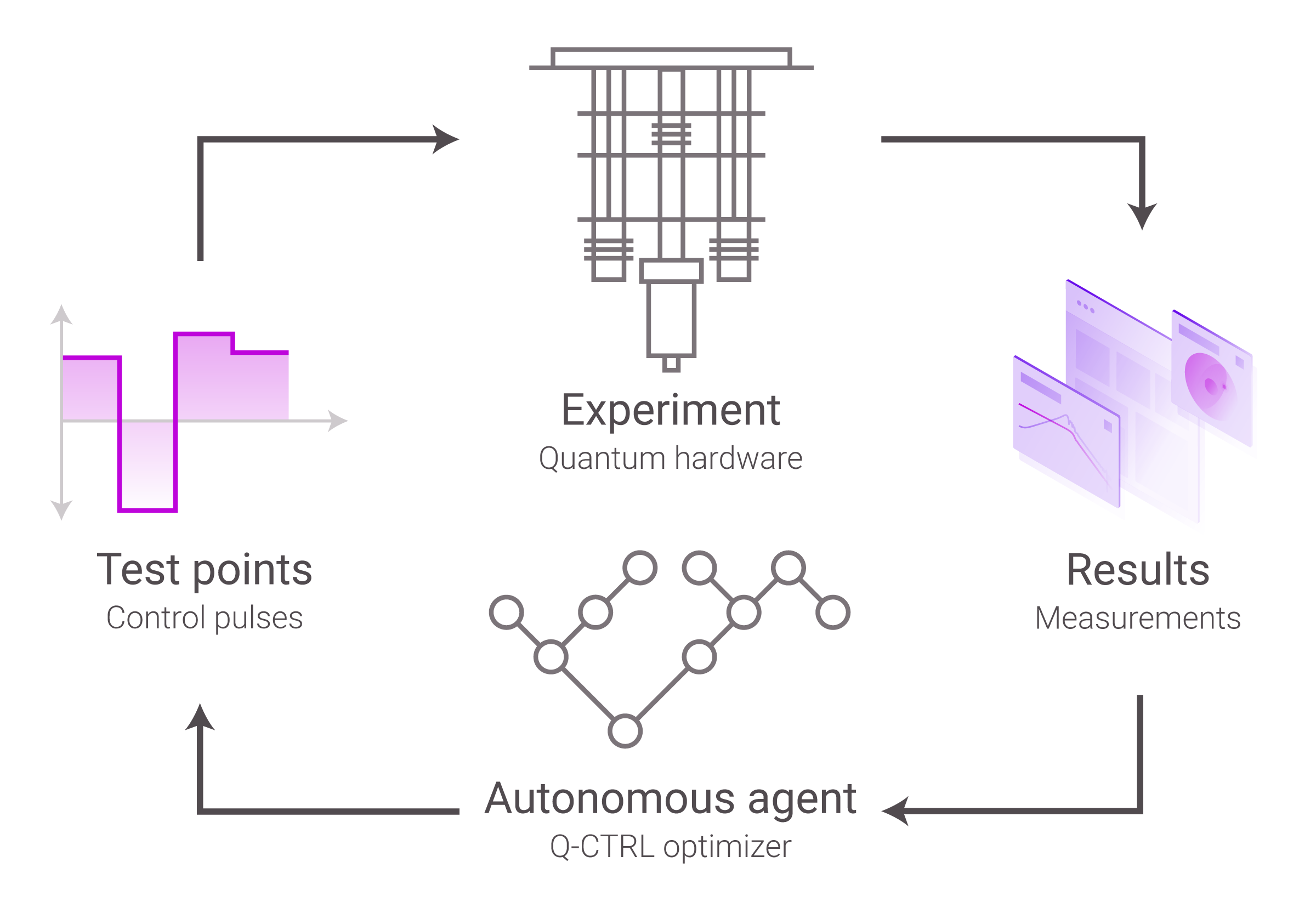## Obtaining an optimal pulse to implement a single qubit gate

Your task is to find an optimal pulse which implements an X gate on a qubit by using Boulder Opal’s closed-loop optimizer. You would usually do this for an experiment, but in this case you will interact with a simulated qubit implemented by the run_experiments function below.

Do not worry about the details in this function, just think of it as a function that interacts with the experiment for which you want to find the optimal gate. It takes in an array of controls, a duration, and a shot_count, and returns an array of measurements on the qubit associated with each control and shot.

In order to obtain the optimal pulse, you will configure the closed-loop optimizer, create functions so that the optimizer and the “experiment” can exchange information, and set up a closed loop for the optimization.

def run_experiments(controls, duration, shot_count):
"""
Simulates a single qubit experiment using Boulder Opal with the given piecewise-constant controls.

Parameters
----------
controls : np.ndarray
The controls to simulate. A 2D NumPy array of (control_count, segment_count) shape
with the per-segment values of each control, as a 2D NumPy array.
duration : float
The duration (in nanoseconds) of the controls.
shot_count : int
The number of shots for which to generate measurements.

Returns
-------
np.ndarray
The qubit measurement results (either 0, 1, or 2) associated to each control
and shot, as an array of shape (len(controls), shot_count).
"""

# Create Boulder Opal graph.
graph = qctrl.create_graph()

# Define simulation parameters and operators.
filter_cutoff_frequency = 2 * np.pi * 0.3  # GHz
segment_count = 128
max_drive_amplitude = 2 * np.pi * 0.1  # GHz
delta = -0.33 * 2 * np.pi  # GHz
big_delta = 0.01 * 2 * np.pi  # GHz
number_operator = graph.number_operator(3)
drive_operator = 0.5 * graph.annihilation_operator(3)
confusion_matrix = np.array(
[[0.99, 0.01, 0.01], [0.01, 0.98, 0.01], [0.0, 0.01, 0.98]]
)

# Retrieve control information.
control_values = controls * max_drive_amplitude

# Define initial state.
initial_state = graph.fock_state(3, 0)

# Construct constant Hamiltonian terms.
frequency_term = big_delta * number_operator
anharmonicity_term = (
delta * (number_operator @ number_operator - number_operator) / 2
)

# Construct filtered drive.
filtered_drive = graph.convolve_pwc(
pwc=graph.pwc_signal(duration=duration, values=control_values),
kernel=graph.sinc_convolution_kernel(filter_cutoff_frequency),
)
drive = graph.discretize_stf(filtered_drive, duration, segment_count)
drive_term = graph.hermitian_part(drive * drive_operator)

# Build Hamiltonian and calculate unitary evolution operators.
hamiltonian = drive_term + frequency_term + anharmonicity_term
unitary = graph.time_evolution_operators_pwc(
hamiltonian=hamiltonian, sample_times=np.array([duration])
)
unitary = unitary[:, -1]

# Evolve initial state and calculate final (normalized) populations.
final_state = unitary @ initial_state[:, None]
populations = graph.abs(final_state) ** 2

# Apply the confusion matrix to the populations.
populations = confusion_matrix @ populations
populations.name = "populations"

# Execute graph and retrieve the populations.
result = qctrl.functions.calculate_graph(
graph=graph, output_node_names=["populations"]
)
populations = result.output["populations"]["value"].squeeze()

# Simulate measurements for each control.
measurements_list = []
for p in populations:
# Sample and store measurements.
measurements = np.random.choice(3, size=shot_count, p=p / np.sum(p))
measurements_list.append(measurements)

return np.array(measurements_list)


### 1. Import libraries and start a Boulder Opal session

Before doing any calculation with Boulder Opal, you always need to import the necessary libraries and start a session.

In this case, import the numpy, matplotlib.pyplot, qctrlvisualizer, and qctrl packages. To learn more about installing Boulder Opal and starting a session, see the Get started guide.

import numpy as np
import matplotlib.pyplot as plt
import qctrlvisualizer

plt.style.use(qctrlvisualizer.get_qctrl_style())

from qctrl import Qctrl

# Start a session with the API.
qctrl = Qctrl()


### 2. Configure closed-loop optimization

#### Configure and initialize the optimizer

Next, you will set up a cross-entropy optimizer to carry out your optimization. You can read our Choosing a control-design (optimization) strategy in Boulder Opal topic to learn more about the optimization routines available in Boulder Opal. Each one of them has their own strengths, and will require a slightly different setup in this step.

Create a qctrl.types.closed_loop_optimization_step.CrossEntropyInitializer object with the elite_fraction of best pulses you want to keep at each iteration, which should be a value smaller than 1. You can also pass it an integer rng_seed for the random number generator that the optimizer will use internally if you want the optimizer to generate deterministic results.

# Create the initializer object for the optimizer.
initializer = qctrl.types.closed_loop_optimization_step.CrossEntropyInitializer(
elite_fraction=0.25, rng_seed=1
)


Finally, pass this initializer object to qctrl.types.closed_loop_optimization_step.Optimizer to set up an automated closed-loop optimization that uses the cross-entropy method with the parameters that you have defined.

# Define state object for the closed-loop optimization.
optimizer = qctrl.types.closed_loop_optimization_step.Optimizer(
cross_entropy_initializer=initializer
)


Now that you have an Optimizer object, you can start setting up the optimization loop.

### 3. Create an optimization loop

#### Create an initial set of results to seed the optimizer

To kickstart the optimization, you need to provide a set of controls and their associated cost. If you had some pulses that you want to refine you could use those, but since you don’t, you can generate a random set of pulses.

Start by defining the parameters for the control that you want to obtain, its number of piecewise-constant segments the control will have and its duration, and the experimental parameters, the number of projective measurements (shots) you want to take after each control and the number of controls you want to test at each optimization iteration. For this last one, make sure that elite_fraction × test_point_count is at least two so the cross-entropy optimizer can advance.

With these parameters, create an array of shape (test_point_count, segment_count) random values for the controls to start the optimization with. You can pass these controls to the experiment (in this case the run_experiment) and obtain their measurements. You can also plot the results of these measurements to have an idea of what they look like.

# Number of controls to retrieve from the optimizer each run.
test_point_count = 16

# Number of segments in each control.
segment_count = 10

# Duration of each control.
duration = 100  # ns

# Number of projective measurements to take after the control is applied.
shot_count = 1000

# Define parameters as a set of controls with piecewise-constant segments.
rng = np.random.default_rng(seed=1)
controls = rng.uniform(0.0, 1.0, size=(test_point_count, segment_count))

# Obtain a set of initial experimental results.
measurements = run_experiments(
controls=controls, duration=duration, shot_count=shot_count
)

Your task calculate_graph (action_id="1139616") has completed.

# Plot distribution of measurements for the initial set of pulses.
fig, ax = plt.subplots(figsize=(20, 5))
c = ax.pcolor(measurements, cmap=plt.get_cmap(lut=3), vmin=0, vmax=2)
ax.set_xlabel("Shot #")
ax.set_yticks(np.arange(test_point_count) + 0.5)
ax.set_yticklabels(np.arange(test_point_count))
ax.set_ylabel("Control #")
cbar = fig.colorbar(
c, ax=ax, ticks=[1 / 3, 1, 5 / 3], label="Measurement results", pad=0.02
)
cbar.ax.set_yticklabels([rf"$|{k}\rangle$" for k in np.arange(0, 3)])
fig.suptitle("Initial measurement results", x=0.45)
plt.show()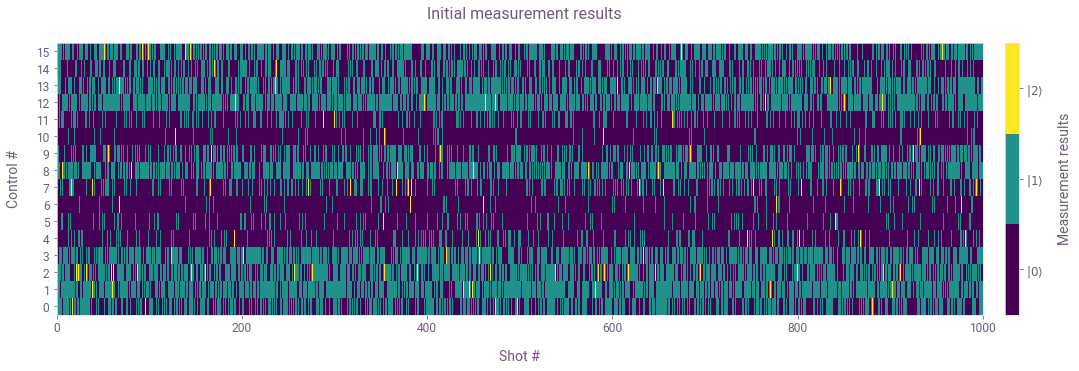The controls that you have generated are random, so we don’t expect to do very well yet. In fact you can see that each control leads to a different distribution of measurements after it’s applied.

#### Create a function to calculate the cost from the experiment results

The function calling the experiment (run_experiments) returns an array with the qubit measurements associated to each control. For each control, the measurements are a list of 0, 1, or 2, corresponding to the measured qubit state after the pulse in a different realization.

From those measurements, you need to define a cost that the optimizer will minimize. As the goal is to perform an X gate on the qubit, you want to maximize the probability of finding the qubit in state $| 1 \rangle$. Thus, define the cost for each control as one minus the probability of finding the qubit in state $| 1 \rangle$.

# Calculate cost from experiment results.
def costs_from_measurements(measurements):
"""
Accepts an array of experiment measurements (from run_experiments)
and return their associated costs.
"""

costs = []
for shots in measurements:
shots_in_one = np.count_nonzero(shots == 1)
fidelity = shots_in_one / len(shots)
costs.append(1 - fidelity)

return costs

costs = costs_from_measurements(measurements)
print("Initial batch of costs:", np.round(costs, 3))

Initial batch of costs: [0.49  0.258 0.379 0.349 0.819 0.882 0.929 0.751 0.357 0.598 0.937 0.755
0.278 0.41  0.614 0.457]


#### Define variables to keep track of the best cost and controls obtained

While the optimizer is running you will need to keep track of what are the lowest cost and best control that you have reached so far. Create variables to store them.

best_cost_overall, best_control_overall = min(
zip(costs, controls), key=lambda params: params
)
print(f"Initial best cost: {best_cost_overall:.3f}")

Initial best cost: 0.258


#### Create function to turn the experimental results into optimizer-friendly inputs

Before creating the main loop, you should define some functions so the optimizer and the experiment can talk to each other, converting the outputs of one into inputs for the other one, and viceversa, as well as a function to calculate the cost for the optimizer from the experiment results.

At each iteration you will need to provide the optimizer with a list of qctrl.types.closed_loop_optimization_step.CostFunctionResult objects for each experiment you have performed in that iteration. Each of those objects needs to contain the values used for the optimizable parameters (the control values) and the cost (infidelity) associated with those parameters.

# Function to organize the experiment results into the optimizer's input format.
def organize_experiment_results(controls, costs):
"""
Accepts an array of controls and their associated costs, and organizes them
in a format accepted by the closed-loop optimizer.
"""

return [
qctrl.types.closed_loop_optimization_step.CostFunctionResult(
parameters=control, cost=cost
)
for control, cost in zip(controls, costs)
]


#### Create function to turn the optimizer outputs into inputs suitable for the experiment

The optimizer will return a Result object which includes the new test points to be passed to the experiment.

The function calling the experiment, run_experiments, accepts the duration (in nanoseconds) and the controls for each experiment. The controls are expected as a 2D array with the values of the pulse (in some arbitrary units) for each pulse and for each piecewise-constant segment.

# Function to organize the optimizer test points into the experiment's input format.
def organize_test_points(test_points):
"""
Accepts a list of test points from the opti
mizer, and organizes them
in a format accepted by the function running the experiment.
"""

return np.array([test_point.parameters for test_point in test_points])


#### Define and execute optimization loop

You now have all of the elements to create a closed optimization loop to obtain the best controls.

You can start the loop by formatting the experiment results (the controls and costs) with organize_experiment_results and passing them to the optimizer function qctrl.functions.calculate_closed_loop_optimization_step. Pass to this function also the optimizer that you have defined above.

From the optimization_result returned from the optimizer you need to take two things. First, its state attribute, with which you create a new qctrl.types.closed_loop_optimization_step.Optimizer object to pass the next call to the optimizer function. Secondly, you need to retrieve the new controls to test suggested from the optimizer from its test_points. You can pass these to the organize_test_points you defined above to obtain the properly formatted controls for the experiment.

After running the experiments with the points suggested by the optimizer, calculate their associated costs and see if any is better than the best one so far (best_cost_overall). You can also print the best cost the optimizer has achieved to see the optimizer’s progress as it’s running, and store it to visualize the convergence of the optimizer after it finishes.

Set up a breaking condition if a desired target_cost is reached. It is also a good idea to set up a maximum number of iterations in case the problem is hard for the optimizer to converge.

max_iteration_count = 20
target_cost = 0.02

# Print the current best cost.
print(f"Initial best cost: {best_cost_overall:.3f}")

# Store the cost history.
best_cost_history = [best_cost_overall]

# Run the optimization loop until the cost (infidelity) is sufficiently small.
for iteration_count in range(max_iteration_count):

# Organize the experiment results into the proper input format.
results = organize_experiment_results(controls, costs)

# Call the automated closed-loop optimizer and obtain the next set of test points.
print("\tRunning optimizer…")
optimization_result = qctrl.functions.calculate_closed_loop_optimization_step(
optimizer=optimizer, results=results, test_point_count=test_point_count
)

# Retrieve the optimizer state and create a new optimizer object.
optimizer = qctrl.types.closed_loop_optimization_step.Optimizer(
state=optimization_result.state
)

# Organize the data returned by the automated closed-loop optimizer.
controls = organize_test_points(optimization_result.test_points)

# Obtain experiment results that the automated closed-loop optimizer requested.
print("\tRunning experiments…")
measurements = run_experiments(
controls=controls, duration=duration, shot_count=shot_count
)

# Obtain the optimization costs from the experiment results.
costs = costs_from_measurements(measurements)

# Record the best results after this round of experiments.
best_cost, best_control = min(zip(costs, controls), key=lambda params: params)

# Compare last best results with best result overall.
if best_cost < best_cost_overall:
best_cost_overall = best_cost
best_control_overall = best_control

# Print the current best cost.
print(f"\nBest cost after {iteration_count+1} iterations: {best_cost_overall:.3f}")

# Store the current best cost.
best_cost_history.append(best_cost_overall)

# Check if desired threshold has been achieved.
if best_cost_overall < target_cost:
print("Target cost reached. Stopping the optimization")
break

Initial best cost: 0.258
Running optimizer…

Running experiments…

Best cost after 1 iterations: 0.125
Running optimizer…

Running experiments…

Best cost after 2 iterations: 0.116
Running optimizer…

Running experiments…

Best cost after 3 iterations: 0.093
Running optimizer…

Running experiments…

Best cost after 4 iterations: 0.036
Running optimizer…

Running experiments…

Best cost after 5 iterations: 0.036
Running optimizer…

Running experiments…

Best cost after 6 iterations: 0.033
Running optimizer…

Running experiments…

Best cost after 7 iterations: 0.033
Running optimizer…

Running experiments…

Best cost after 8 iterations: 0.027
Running optimizer…

Running experiments…

Best cost after 9 iterations: 0.027
Running optimizer…

Running experiments…

Best cost after 10 iterations: 0.026
Running optimizer…

Running experiments…

Best cost after 11 iterations: 0.022
Running optimizer…

Running experiments…

Best cost after 12 iterations: 0.019
Target cost reached. Stopping the optimization


You can see that as the iterations progress, the best cost found by the optimizer gets smaller until it reaches the target cost and the optimization is stopped.

### Retrieve the best results obtained by the optimizer

You can now print the best cost and controls the optimizer has achieved and visualize them with the plot_controls function from the Q-CTRL Visualizer.

# Print final best cost.
print(f"Best cost reached: {best_cost_overall:.3f}")

# Print and plot controls that correspond to the best cost.
print(f"Best control values: {np.round(best_control_overall, 3)}")

qctrlvisualizer.plot_controls(
figure=plt.figure(),
controls={
r"$\Omega(t)$": qctrl.utils.pwc_arrays_to_pairs(duration, best_control_overall)
},
two_pi_factor=False,
unit_symbol=" a.u.",
)
plt.suptitle("Best control")
plt.show()

Best cost reached: 0.019
Best control values: [0.577 0.744 0.335 0.688 0.67  0.287 0.145 0.298 0.353 0.342]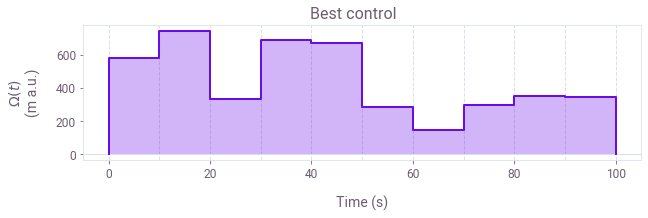Similarly as you did before running the optimizer, you can also visualize the distribution of measurements for the final set of pulses suggested by the optimizer.

# Plot distribution of measurements for the final set of pulses.
fig, ax = plt.subplots(figsize=(20, 5))
c = ax.pcolor(measurements, cmap=plt.get_cmap(lut=3), vmin=0, vmax=2)
ax.set_xlabel("Shot #")
ax.set_yticks(np.arange(test_point_count) + 0.5)
ax.set_yticklabels(np.arange(test_point_count))
ax.set_ylabel("Control #")
cbar = fig.colorbar(
c, ax=ax, ticks=[1 / 3, 1, 5 / 3], label="Measurement results", pad=0.02
)
cbar.ax.set_yticklabels([rf"$|{k}\rangle$" for k in np.arange(0, 3)])
fig.suptitle("Final measurement results", x=0.45)
plt.show()

print("Final batch of costs:", np.round(costs, 3))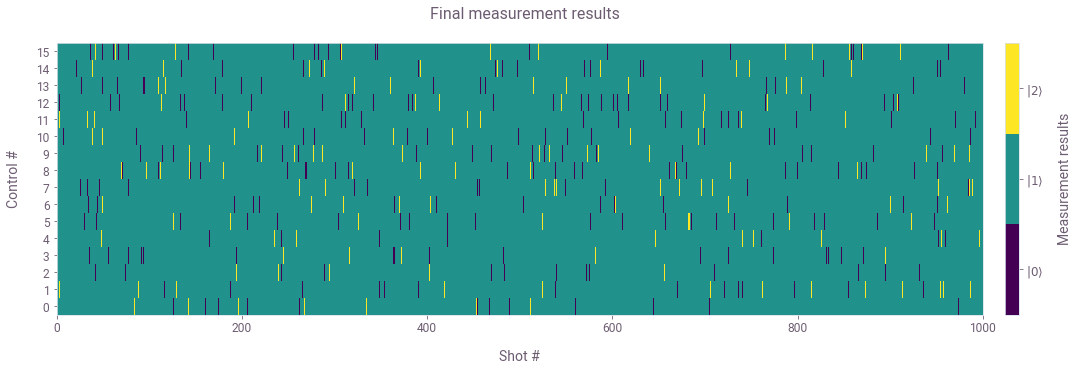Final batch of costs: [0.021 0.03  0.02  0.022 0.019 0.029 0.029 0.025 0.036 0.035 0.025 0.034
0.038 0.026 0.029 0.033]


You can observe how with most of the pulses suggested by the optimizer the atom ends up in state $|1\rangle$ as expected.

### Visualize the convergence of the optimizer

You can now also plot the cost history during the optimization, and see its decrease until it crosses the target threshold. You can easily do this with the plot_cost_history function from the Q-CTRL Visualizer.

qctrlvisualizer.plot_cost_history(plt.figure(), best_cost_history, y_axis_log=False)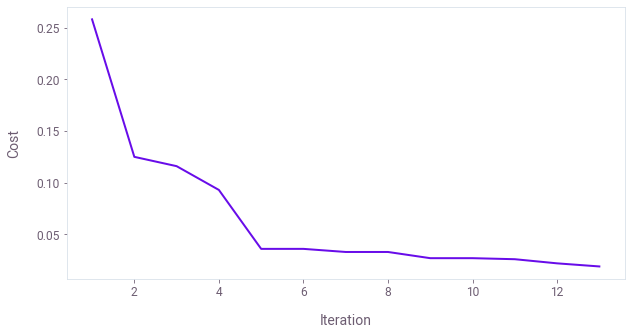This concludes the tutorial. Congratulations on optimizing your first control!

You can now try to change the optimization loop you are running here by changing the optimization parameters or using a completely different optimizer. You can also play around with the function simulating the experiment and, for instance, make it harder or easier for the optimizer to find solutions by changing the confusion matrix or the anharmonicity in the system.

Our user guides offer more examples and problems to which you can apply this automated optimization tool. In particular, you might be interested in reading about obtaining optimal controls with other closed-loop optimization routines, calibrating control hardware, or using the open-source M-LOOP package to integrate the experiment to Boulder Opal closed-loop optimizer.